# RD Sharma Class 10 Solutions Chapter 1 Real Numbers Ex 1.6

## RD Sharma Class 10 Solutions Chapter 1 Real Numbers Ex 1.6

These Solutions are part of RD Sharma Class 10 Solutions. Here we have given RD Sharma Class 10 Solutions Chapter 1 Real Numbers Ex 1.6

Other Exercises

Question 1.
Without actually performing the long division, state whether the following rational numbers will have a terminating decimal expansion or a non-terminating repeating decimal expansion.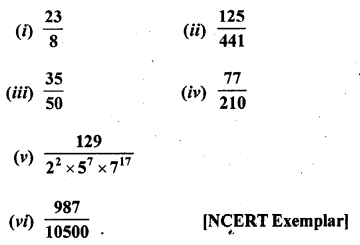Solution: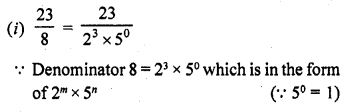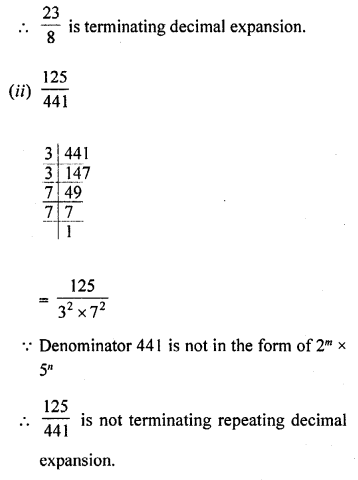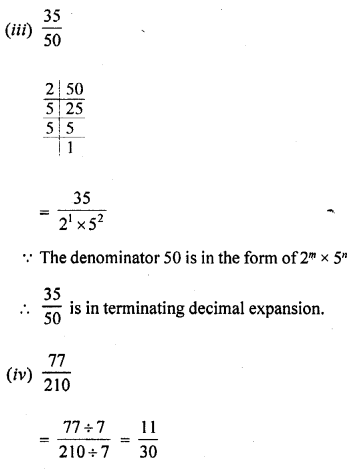Since, the denominator is of the form 2m x 5n, the rational number has a terminating decimal expansion.

Question 2.
Write down the decimal expansions of the following rational numbers by writing their denominators in the form 2m x 5n, where m and n are non-negative integers: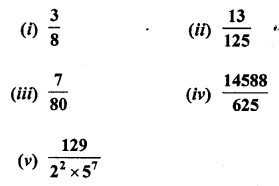Solution: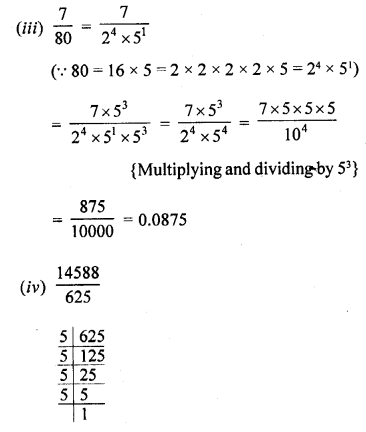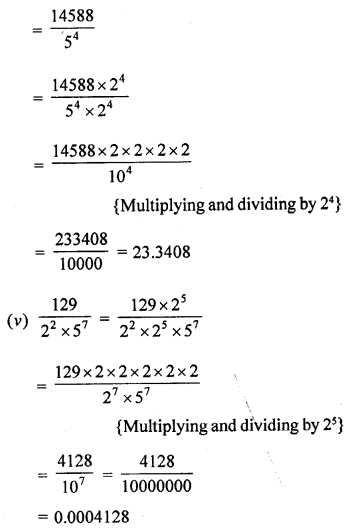Question 3.
Write the denominator of the rational number $$\frac { 257 }{ 5000 }$$ in the form 2m x 5n , where m, n are non-negative integers. Hence, write the decimal expansion, without actual division.
Solution: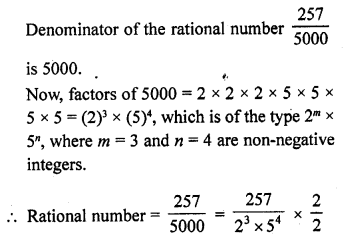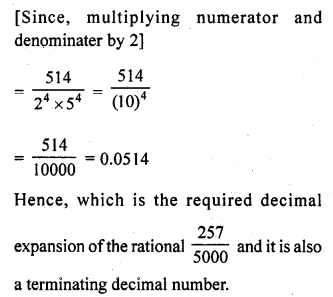Question 4.
What can you say about the prime factorisations of the denominators of the following rationals :
Solution:
(i) 43.123456789
This decimal fraction is terminating Its denominator will be factorised in the form of 2m x 5n where m and n are non-negative integers.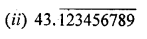This decimal fraction is non-terminating repeating decimals.
The denominator of their fraction will be not in the form of 2m x 5n where m and n are non-negative integers.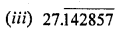This decimal fraction is terminating
Its denominator will be factorised in the form of 2m x 5n where m and n are non-negative integers
(iv) 0.120120012000120000 ………..
This decimal fraction in non-terminating non recurring
Its denominator will not be factorised in the form of 2m x 5n where m and n are non negative integers

Question 5.
A rational number in its decimal expansion is 327.7081. What can you say about the prime factors of q, when this number is expressed in the form $$\frac { p }{ q }$$ ? Give reasons. [NCERT Exemplar]
Solution:
327.7081 is terminating decimal number. So, it represents a rational number and also its denominator must have the form 2m x 5n.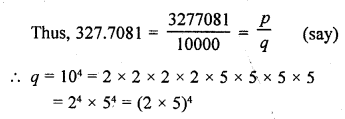Hence, the prime factors of q is 2 and 5.

Hope given RD Sharma Class 10 Solutions Chapter 1 Real Numbers Ex 1.6 are helpful to complete your math homework.

If you have any doubts, please comment below. Learn Insta try to provide online math tutoring for you.